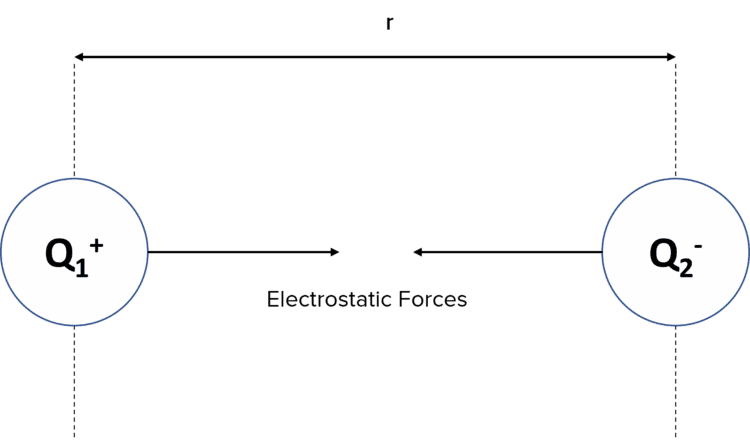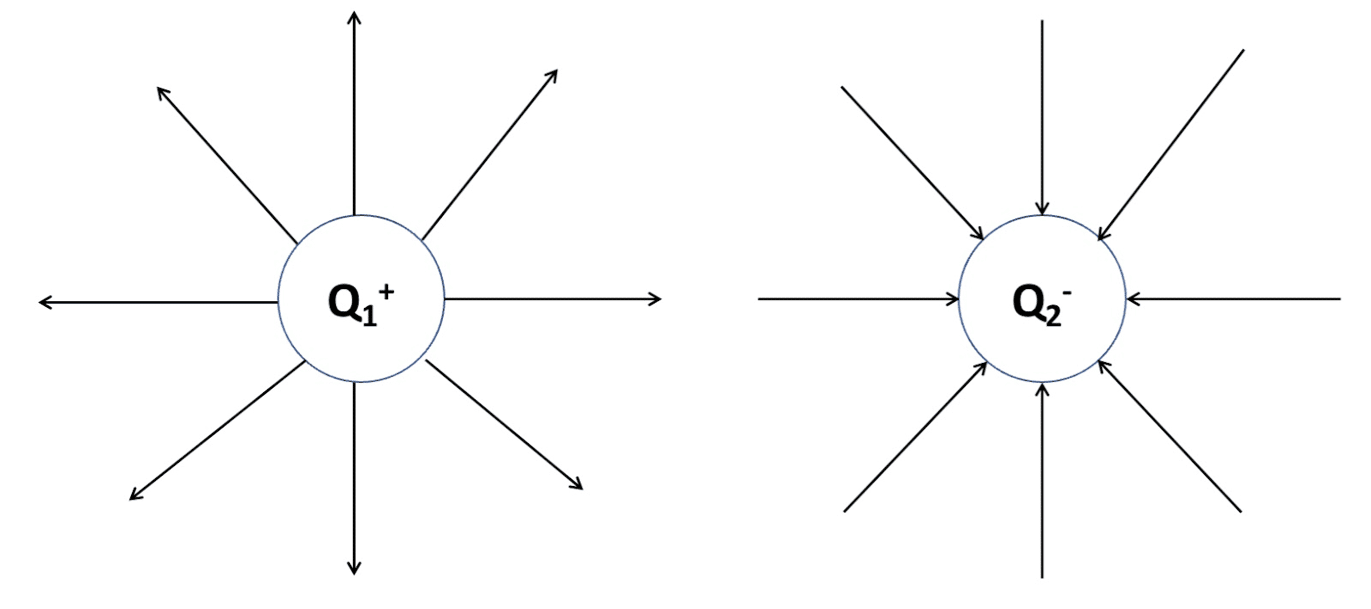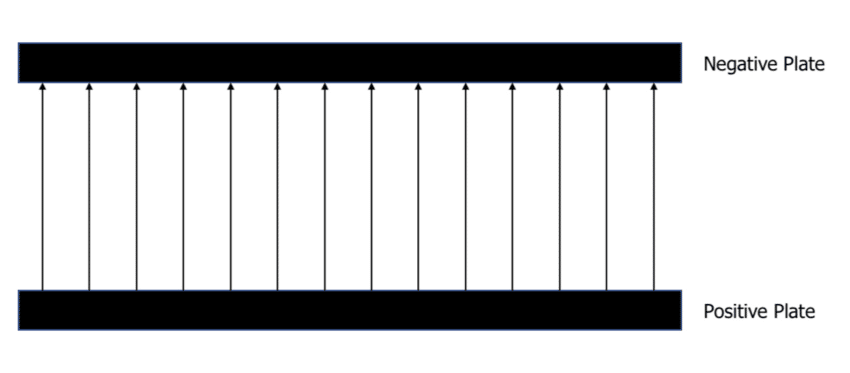# Coulomb's Law

A LevelAQA

## Coulomb’s Law

We should recall from GCSE level that charges interact. Like charges repel and opposite charges attract. This section takes this idea a step further allowing us to calculate the force of attraction or repulsion using an equation called Coulomb’s Law.

## Coulombs Law

All charged particles produce an electric field around them. As with any force field, the electric field will exert a force on any object within the field.

The force can be an attractive force if between two opposite charges or a repulsive force if between two like charges.Coulomb’s Law describes how two charged particles interact with each other if they are within the electric field of one another. It states that the electrostatic force is proportional to the product of the two charges and inversely proportional to the square of the distance between the charges. We consider the charge to be at the centre of a charged sphere.

$E \propto Q_1 Q_1$ and $E \propto \dfrac{1}{r^2}$

This is represented in the equation for Coulomb’s Law:

$F = \dfrac{Q_1 Q_2}{4 \pi \epsilon_0 r^2}$

• $F$ is the force between the two charges in Newtons $\left(\text{N}\right)$.
• $Q_1$ and $Q_2$ are the charges in Coulombs $\left(\text{C}\right)$.
• $\epsilon_0$ is the permittivity of free space, $8.85 \times 10^{-12} \: \text{Fm}^{-1}$. This is given on the formula sheet.
• $r$ is the distance between the centre of the two charges in metres $\left(\text{m}\right)$.

When using Coulomb’s Law, we treat air as a vacuum, hence why we use the permittivity of free space $\left( \epsilon_0\right)$

Example: Two protons are $2 \: \text{mm}$ apart. Calculate the electrostatic force of repulsion between the protons given that the charge of a proton is $1.60 \times 10^{-19} \: \text{C}$.

[3 marks]

$F = \dfrac{Q_1 Q_2}{4 \pi \epsilon_0 r^2}$

$F = \dfrac{1.6 \times 10^{-19} \times 1.6 \times 10^{-19}}{4 \pi 8.85 \times 10^{-12} \times 0.002^2}$

$F = 5.75 \times 10^{-23} \: \text{N}$

A LevelAQA

## Electric Fields

By convention, the direction of electric field lines are always drawn away from the positive charge and towards the negative charge.As the field lines are not evenly distributed at all points within the field, radial fields like those above are considered non-uniform.

An example of a uniform electric field can be found between positively charged and negatively charged plates. Remember, the field lines will always flow from positive to negative:At all points between the plates, the electric field lines are equally spaced. Therefore, the electric field strength is equal at all points.

A LevelAQA

## Coulomb's Law Example Questions

The electrostatic force is proportional to the product of the two charges and inversely proportional to the square of the distance between the charges.

OR

$F = \dfrac{Q_1 Q_2}{4 \pi \epsilon_0 r^2}$

Where $F$ is the force between the two charges,  $Q_1$ and $Q_2$ are the charges, $\epsilon_0$ is the permittivity of free space $\left(8.85 \times 10^{-12} \: \text{Fm}^{-1}\right)$ and $r$ is the distance between the centre of the two charges.

\begin{aligned} F &= \dfrac{Q_1 Q_2}{4 \pi \epsilon_0 r^2} \\ \\ F &= \dfrac{\left(-1.60 \times 10^{-19}\right)^2}{\left(4 \times \pi \times 8.85 \times 10^{-12} \times 0.001^2\right)} \\ \\ F &= 2.3 \times 10^{-22} \: \text{N} \end{aligned}

The field lines would vary in distance apart.## You May Also Like...### MME Learning Portal

Online exams, practice questions and revision videos for every GCSE level 9-1 topic! No fees, no trial period, just totally free access to the UK’s best GCSE maths revision platform.

£0.00